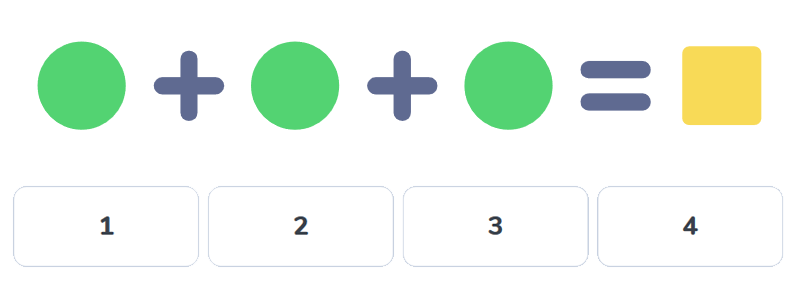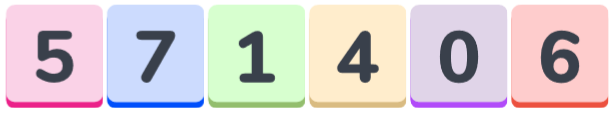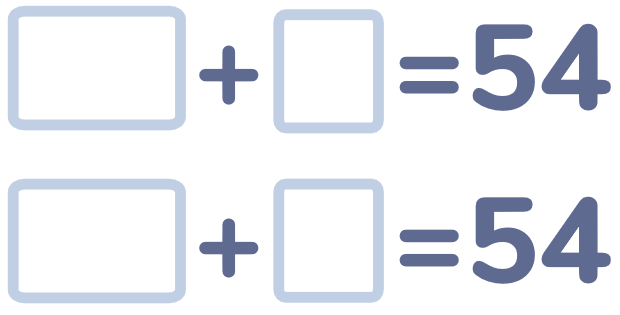Brain teasers / Mathematical

3500+ fun and challenging puzzles with solutions for kids 5-12 years old!

## Learn math in a playful way

with Number Puzzles and Math Riddles by LogicLike!

## Math Puzzles and Problems with Numbers

We update collection of puzzles and riddles every week!

## Easy math puzzles for kids

If you rotate this number by 180 degrees clockwise, it will become smaller by 3.Two people are walking into town. Another two people are going the other way.
How many people are going into town altogether?

## Number Puzzles

There are 10 fingers on two hands.
How many fingers are there on ten hands?Score 8 points.

Look for similar tasks on the page Number Puzzles.

## Arithmetic puzzle for schoolchildrenWhat digit hides behind a triangle?Choose all the digits that a circle can be equal to.## Tricky math riddleWhich weighs less:
1 kg of nails or 1 kg of feathers?Ten cars were brought to the showroom.
All but nine of them were bought.
How many cars were left?

## Challenging math puzzlesRemove 3 digits so that the other digits form the biggest three-digit number possible.The sum of two numbers is 54.
The smaller number can be found by crossing out a digit out of the bigger number.